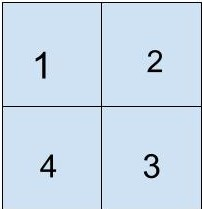# Ninja’s 3D structures

Posted: 18 Apr, 2021
Difficulty: Moderate

## PROBLEM STATEMENT

#### Note:

``````The bottom face of each shape counts toward its surface area.
``````

#### Example

``````Let say ‘N’ = 2 and ‘GRID’ = [ [1,2] , [ 4, 3] ]. The the grid will look like this
````````````Here, Ninja has kept 1 cube at ‘GRID’, 2 cubes at ‘GRID’, 4 cubes at ‘GRID’ and three cubes at ‘GRID’.

If we have to find the total surface area of this structure then, we will have to subtract the total surface area that has glued up.

After subtracting, the total surface area of the structure made by Ninja would be 34.
``````
##### Input Format:
``````The first line contains a single integer ‘T’ representing the number of test cases.

The first line of each test case will contain a single integer ‘N’ which denotes the grid’s size.

The next ‘N’ lines contain the ‘N’ space-separated integers which denote the value of ‘GIRD[i][j]’.
``````
##### Output Format:
``````For each test case, return the total surface area of the structure.
``````
##### Note:
``````You don’t need to print anything; It has already been taken care of. Just implement the given function.
``````
##### Constraints:
``````1 <= T <= 50
1 <= N <= 100
1 <= GRID[ i ][ j ] <= 50

Time limit: 1 sec
``````Approach 1

The basic idea is to calculate the total surface area by each cell in the grid. For each tower, its surface area is 4*v + 2.

However, 2 adjacent towers will hide the area of the connected part. The hidden part is min(v1, v2), and we need to just subtract twice of this hidden area (as the hidden area will contain faces of both the adjacent cubes).

The steps are as follows:

• Initialize a variable 'RES' to store the result; this will be of type int. Initially, its value will be 0.
• Iterate  0 to ‘N’(say iterator = ‘i’ ).
• Iterate  from 0 to ‘N’.(say iterator = ‘j’)
• If ‘GRID[i][j]’ is equals to 1 then increase ‘RES’ by 'GRID[i][j]’*4 + 2 .
• If ‘i’ is 1 then decrease two times the minimum of “GRID[i][j]” and “GRID[i-1][j]” from ‘RES’.
• If ‘j’ is 1 then decrease two times the minimum of “GRID[i][j]” and “GRID[i][j-1]” from ‘RES’.
• Return ‘RES’